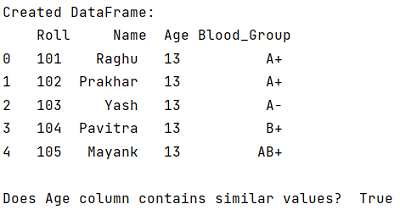# Check if all values in dataframe column are the same

Given a pandas dataframe, we have to check if all values in dataframe column are the same.
Submitted by Pranit Sharma, on September 20, 2022

Pandas is a special tool that allows us to perform complex manipulations of data effectively and efficiently. Inside pandas, we mostly deal with a dataset in the form of DataFrame. DataFrames are 2-dimensional data structures in pandas. DataFrames consist of rows, columns, and data.

## Checking if all values in dataframe column are the same

Suppose, we have a column for some student of the same class, the DataFrame will have the following columns:

• Roll Number
• Name
• Age
• Blood Group
• Marks

We need to check if all the students are of the same age or not or we need to check that does the Age column contains the same values or not.

For this purpose, we will first convert the column into a NumPy array and then we will compare the first element of this array with all the other elements.

Let us understand with the help of an example,

## Python code to check if all values in dataframe column are the same

```# Importing pandas package
import pandas as pd

# Creating dictionary
d = {
'Roll':[101,102,103,104,105],
'Name':['Raghu','Prakhar','Yash','Pavitra','Mayank'],
'Age':[13,13,13,13,13],
'Blood_Group':['A+','A+','A-','B+','AB+']
}

# Creating DataFrame
df = pd.DataFrame(d)

# Display Original DataFrames
print("Created DataFrame:\n",df,"\n")

# Checking the Age column
arr = df['Age'].to_numpy()
print(arr)
print("Does Age column contains similar values? ",(arr == arr).all())
```

Output:What's New (MCQs)

Top Interview Coding Problems/Challenges!

Languages: » C » C++ » C++ STL » Java » Data Structure » C#.Net » Android » Kotlin » SQL
Web Technologies: » PHP » Python » JavaScript » CSS » Ajax » Node.js » Web programming/HTML
Solved programs: » C » C++ » DS » Java » C#
Aptitude que. & ans.: » C » C++ » Java » DBMS
Interview que. & ans.: » C » Embedded C » Java » SEO » HR
CS Subjects: » CS Basics » O.S. » Networks » DBMS » Embedded Systems » Cloud Computing
» Machine learning » CS Organizations » Linux » DOS
More: » Articles » Puzzles » News/Updates

© https://www.includehelp.com some rights reserved.